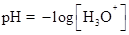Chapter 16.7, Problem 16.4CYU

Chapter
Section
Textbook Problem

A solution prepared from 0.055 mol of butanoic acid and sufficient water to give 1.0 L of solution has a pH of 2.72. Determine Ka for butanoic acid. The acid ionizes according to the balanced equationCH3CH2CH2CO2H(aq) + H2O(l) ⇄ H3O+(aq) + CH3CH2CH2CO2−(aq)

Interpretation Introduction

Interpretation:

The value of Ka has to be calculated for butanoic acid.

Concept introduction:

The pH is a unit of measure which describe the degree of alkanity or acidity of a solution.The acid dissociation constant (Ka) is the equilibrium constant of the dissociation reaction of an acid.

For an aqueous solution the general form of an acid dissociation equilibrium reaction is written as;

HA + H2 A+ H3O+

Explanation

Given:

Concentration of butanoic acid is 0.055molL1 and the solution has pH value 2.72.

The balanced equation of butanoic acid is given below.

CH3CH2CH2CO2H(aq)+H2O(l)H3O++CH3CH2CH2CO2(aq)

Expression for acid dissociation constant is written as,

Ka=[H3O+][CH3CH2CH2CO2][CH3CH2CH2CO2H]

Concentration of [H3O+] is calculated below.

[H3O+]=10pH= 102.72= 1.905×103

Therefore concentration of hydronium ion is 1.905×103 M.

On substituting those values in ICE table (1).

EquationCH3CH2CH2CO2H(aq)+H2O(aq)H3O+(aq)+CH3CH2CH2CO2(aq)Initial(M)0

Still sussing out bartleby?

Check out a sample textbook solution.

See a sample solution

The Solution to Your Study Problems

Bartleby provides explanations to thousands of textbook problems written by our experts, many with advanced degrees!

Get Started

How do eating disorders affect health?

Understanding Nutrition (MindTap Course List)

Why do biologists often use model organisms in their research?

Biology: The Dynamic Science (MindTap Course List)

What is the difference between pyranose and furanose rings?

Chemistry for Today: General, Organic, and Biochemistry